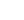# Trigonal Planar Molecular GeometryAX3Shape: trigonal planar Steric Number: 3 Lone Pairs: 0 Polar/NonPolar: NonPolar Hybridization: sp2 Examples: BF3, CO32-

NOTES: This molecule is made up of 3 equally spaced sp2 hybrid orbitals arranged at 120o angles. The shape of the orbitals is planar triangular. Since there is an atom at the end of each orbital, the shape of the molecule is also planar triangular.

## Boron Hydride

An example of trigonal planar electron pair geometry and molecular geometry is BH3. This molecule is electron deficient and does not follow the octet rule because it has only 6 valence electrons. The hydrogen atoms are as far apart as possible at 120o. This is trigonal planar geometry. The molecule all in a plane and is two dimensional.This molecule exists in a gaseous state in only minute quantities under specialized conditions as an intermediate in the making of other boron hydride type molecules.## Formaldehyde

In this example, H2CO, the Lewis diagram shows carbon at the center with no lone electron pairs. The carbon and and the oxygen are bonded through a double bond which counts as "one electron pair". Hence the molecule has three electron pairs and is trigonal planar. Formaldehyde is the simplest member of a class of organic compounds called aldehydes. These compounds have the structural component of the carbon double bond oxygen and at least one hydrogen atom and are always in the trigonal planar format geometry.

Formaldehyde or methanal is a water soluble gas. A 37% solution is water, known as formalin, is a biological preservative and in used in embalming fluids.## Carbonate Ion

In this example, CO32-, the Lewis diagram shows carbon at the center with no lone electron pairs.

The Lewis diagram is as follows:
C = 4 e-
O = 6e- x 3 = 18e-
2- charge = 2e-
Total electrons = 24

One double bond is needed to give carbon an octet. The carbon and oxygen are bonded through a double bond which counts as "one electron pair" and two single bonded oxygens. Hence the molecule has three electron pairs and is trigonal planar geometry. Carbonate ion is present in limestone as calcium carbonate. A form of it, bicarbonate, is in baking soda and baking powder.